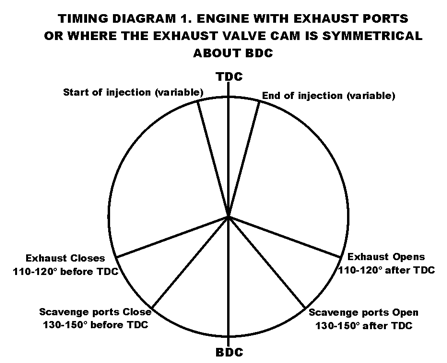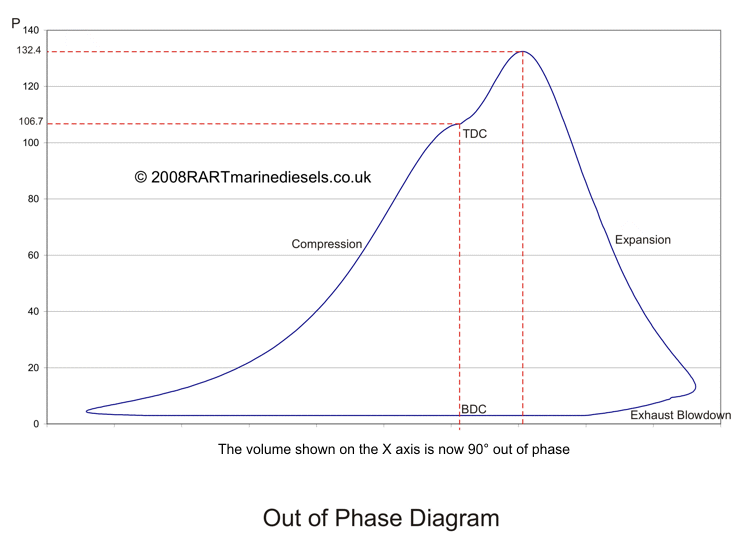Operational Theory

The Actual Diesel Engine Cycle

 ****JavaScript based drop down DHTML menu generated by NavStudio. (OpenCube Inc. - http://www.opencube.com)****

The ideal cycles are theoretical only, and do not show what actually happens inside the cylinder of a large marine diesel engine.

For simplicity and because of its close relationship with the ideal cycles, the following describes a two stroke cycle:Starting at bottom dead centre, the exhaust valve is open and the scavenge ports are uncovered. Air is flowing into the cylinder, pushing out or "scavenging" the remaining exhaust gas.

As the piston moves up the cylinder, the scavenge ports are closed off by the piston. The angle at which this occurs varies but is about 140° BTDC. (Before Top Dead Centre).

The exhaust valve then closes. Again this angle is variable but is about 120° BTDC.

Compression now starts. However it is not adiabatic, where no heat is gained and lost to the liner, piston and cylinder head but polytropic (literally, many temperatures). The index of compression, 'n' is variable but for this example is taken as 1.35.

Although the air inlet to the engine is maintained at about 45 -50°, The air inside the cylinder will have gained heat from the surroundings when the engine is at operating temperature, and at the start of compression is about 75°.

At about 6° BTDC, fuel injection will commence, and will continue through untill about 10° ATDC (After Top Dead Centre), depending on engine load..

The fuel will heat up and start to burn just as the piston comes over TDC (Top Dead Centre). Pressure will rise in the cylinder quickly, reaching a maximum at between 12 and 15° ATDC. The piston is driven down by the rapidly expanding gases, converting the energy in the burning fuel into work.

The length of time the fuel burns for again is variable. For this model it is until 33° ATDC when expansion again follows a polytropic curve with the index 'n' here set at 1.23.

The pressure then drops in the cylinder as the piston moves down the cylinder until the exhaust valve opens (120°ATDC), when the pressure is about 9 bar.

During exhaust blowdown, pressure drops rapidly in the cylinder until the scavenge ports open (140° ATDC) and the pressure drops to scavenge pressure.

Note pressures quoted are absolute: i.e gauge pressure + atmospheric pressure (1bar).

It is possible to model the cycle on a spreadsheet, and to calculate the Mean Indicated Pressure (MIP), Indicated Work and Indicated Power (IP).Because the diagram is a pV curve, the area  enclosed by the diagram is indicative of the work done during the cycle. To calculate the area of the diagram, I subtracted the area under the compression line (i.e BDC - TDC) from the area under the epansion line (i.e TDC - BDC). The area under the polytropic compression and expansion curve are easily calculated because they follow the law pVn=C. Although there is no set equation for the fuel burning curve or the exhaust blowdown curve, the Exel spreadsheet calculated equations which gave a good representation. By integrating the equation between the required values it was possible to calculate the area (and therefore the work done) under those curves. The results can be seen in the table on the diagram.

If the work done per cycle (revolution) is divided by the swept volume then the Mean Indicated Pressure is obtained. In this case it is just over 16.3 bar. When calculating the Indicated Power for a cylinder on an actual engine, it is the Mean Indicated Pressure that needs to be obtained from the indicator diagram. If the Mean Indicated Pressure is multiplied by the swept volume of the cylinder (a known quantity), then the work done/cycle is obtained.

As stated earlier, the air in the cylinder is about 75°C at start of compression. The temperature at the start of fuel injection can be calculated using PV/T = Constant. This gives a temperature of 593° at 6° BTDC and 611° at TDC. The temperature rises rapidly during combustion to about 1500°C depending on the type of fuel.

Because the piston displacement is very small per degree of crank angle around BDC and TDC, and largest at about 70°ATDC (see Piston displacement page) the indicator diagram is different from the one obtained when using crank angle on the x axisThe crank angle/pressure diagram is often used by computer packages which calculate the MIP and IP by measuring the pressure in the engine cylinders using a pressure transducer, whilst at the same time measuring the crank angle using a pick up on the flywheel. However these diagrams tend to measure the crank angle from 0 - 360° and so look like the diagram below.

For examples of these computer drawn indicator cards go to the members section.Because the computer program will have been programmed with the engine bore, stroke and conrod length, it can calculate the MIP and IP from the pressure recorded for each crank angle, which it measures every 0.25°.

Another diagram which can be drawn is the out of phase diagram. In this the crank angle is offset by 90°If the volume on the x axis is moved 90° out of phase with the pressure, (so that at the pressure reached at TDC, the volume is recorded as that at 90° ATDC, when the piston has reached 90°ATDC the volume is recorded as that at BDC, and when the piston has reached BDC the volume is recorded as that of 90°ABDC etc), this will amplify in a horizontal plane what is happening to the pressure in the cylinder allowing faults to be diagnosed.

IN THE MEMBERS SECTION:

DHTML Menu / JavaScript Menu Powered By OpenCube